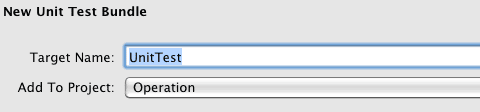## 2011年4月12日 星期二

### [轉] 單元測試 Unit Test 簡單設置

from : http://www.furnace.idv.tw`RectangleTest.h `` #import #import @interface RectangleTest : SenTestCase { } ``@end ` `RectangleTest.m `` #import "RectangleTest.h" @implementation RectangleTest `` @end``RectangleTest.h `` #import `` #import `` #import "Rectangle.h" `` @interface RectangleTest : SenTestCase { Rectangle *theRect; } ``- (void)testArea; ``@end `

`RectangleTest.m `` #import "RectangleTest.h" `` @implementation RectangleTest `` //setUp函式寫入測試之後的環境初始化 ``- (void)setUp ``{ `` theRect = [[[Rectangle alloc]initWithHeight:8 andWeight:25]retain]; `` //建立失敗回傳Could not create test subject. `` STAssertNotNil(theRect, @"Could not create test subject."); ``} `` //tearDown函式寫入測試之後的物件釋放 ``- (void)tearDown ``{ `` [theRect release]; ``} ```` ```` //測試的功能前面加入test字樣 ``- (void)testArea ``{ `` int expected = 200; `` int result = theRect.area; `` //測試兩數值必須相等 `` STAssertEquals(expected, result,@"We expect %d, but it was %d", expected, result); ``}`` @end`

STAssert 能判斷的東西當然不只上述這些，有興趣的讀者可以自行實驗。